# Multiplying 2 digit by 1 digit number

Multiplying 2 digit by 1 digit number – Question 1

Multiply :
13 x 2 = ?

Explanation

Step I: Multiply 3 in ones place by 2
3 x 2 = 6
Write 6 in ones place.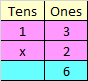Step II: Multiply 1 in tens place by 2
1 x 2 = 2
Write 2 in tens place.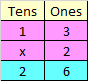Hence, 13 x 2 = 26

Multiplying 2 digit by 1 digit number – Question 2

Multiply :
23 x 3 = ?

Step I: Multiply 3 in ones place by 3
3 x 3 = 9
Write 9 in ones place.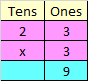Step II: Multiply 2 in tens place by 3
2 x 3 = 6
Write 6 in tens place.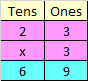Hence, 23 x 3 = 69

Multiplying 2 digit by 1 digit number – Question 3

Multiply :
26 x 3 = ?

Explanation

Step I: Multiply 6 in ones place by 3
6 x 3 = 18
Since, the number is more than 9, we need to carry over 1 to the tens place.
Write 8 at ones place.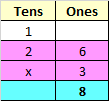Step II: Multiply 2 in tens place by 3
2 x 3 = 6
Add the carry over of 1 to 6
6 + 1 = 7
Write 7 in tens place.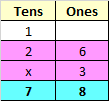Hence, 26 x 3 = 78

Multiplying 2 digit by 1 digit number – Question 4

Multiply :
46 x 2 = ?

Explanation

Step I: Multiply 6 in ones place by 2
6 x 2 = 12
Since, the number is more than 9, we need to carry over 1 to the tens place.
Write 2 at ones place.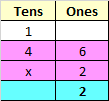Step II: Multiply 4 in tens place by 2
4 x 2 = 8
Add the carry over of 1 to 8
8 + 1 = 9
Write 9 in tens place.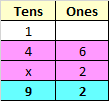Hence, 46 x 2 = 92